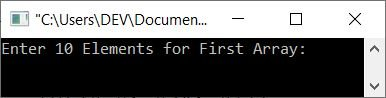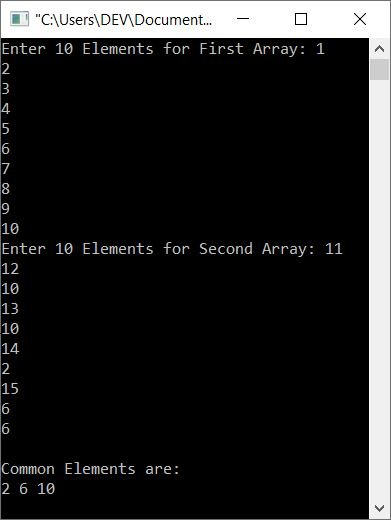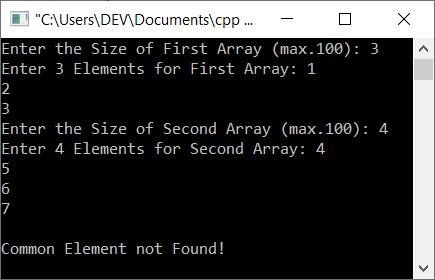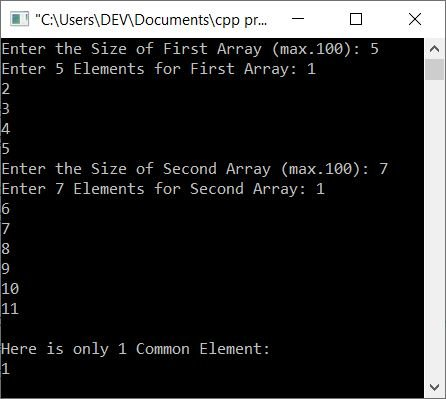# C++ Program to Find and Print Common Elements between Two Arrays

This article deals with some programs in C++ that are used to find and print all the common elements present between two given arrays given by the user at run-time.

For example, if the two given arrays are:

```arr1 = 10, 20, 30, 40, 50, 60, 70, 80, 90, 100
arr2 = 10, 34, 10, 67, 54, 90, 84, 39, 30, 54```

then the common elements between these two arrays are 10, 90, and 30. Because these three elements are available in both arrays.

## Find the Common Elements in Two Arrays

The question is, "Write a program in C++ that receives any two arrays from the user at run-time and finds and prints all the common elements between the two arrays." Here is its answer:

```#include<iostream>

using namespace std;

int main()
{
int arr1, arr2, arr3, i, j, k=0, x, tot;
cout<<"Enter 10 Elements for First Array: ";
for(i=0; i<10; i++)
cin>>arr1[i];
cout<<"Enter 10 Elements for Second Array: ";
for(i=0; i<10; i++)
cin>>arr2[i];

// Finding and storing common elements
for(i=0; i<10; i++)
{
for(j=0; j<10; j++)
{
if(arr1[i]==arr2[j])
{
tot = 0;
for(x=0; x<k; x++)
{
if(arr1[i]==arr3[x])
tot++;
}
if(tot==0)
{
arr3[k] = arr1[i];
k++;
}
}
}
}

// Printing common elements
cout<<"\nCommon Elements are:\n";
for(i=0; i<k; i++)
cout<<arr3[i]<<" ";

cout<<endl;
return 0;
}```

The snapshot given below shows the initial output produced by the above C++ program on finding and printing all the common elements between two given arrays by the user at run-time:Now supply the input, say 1, 2, 3, 4, 5, 6, 7, 8, 9, 10 as ten elements for the first array and 11, 12, 10, 13, 10, 14, 2, 15, 6, 6 as ten elements for the second array. Here is the sample run based on exactly these inputs:The above program is created in such a way that:

• First, all 10 elements are received and stored in array arr1[]
• Second, all 10 elements are received and stored in the array arr2[]
• Now I've compared the element at the very first (or 0th) index in arr1[] with each and every element of the second array, that is, arr2[].
• If any match is found, then the element gets initialized or stored in the third array named arr3[].
• Then I've compared the element at the second (or first) index in arr1[] with each and every element of the second array.
• And again, if a match is found, then the element gets initialized to arr3[].
• In this way, the third array arr3[] holds only the common elements between the given two arrays.

### Find Common Elements Between Two Arrays of Any Size

This is a modified version of the previous program. This program allows the user to define the size of both arrays. Also, this program prints the message according to the output. That is, if no common elements are found between two given arrays, then the program prints the message "Common Element Not Found!" This provides a better user experience.

```#include<iostream>

using namespace std;

int main()
{
int a, b, c, i, j, k=0;
int a_size, b_size, x, cnt=0;
cout<<"Enter the Size of First Array (max.100): ";
cin>>a_size;
cout<<"Enter "<<a_size<<" Elements for First Array: ";
for(i=0; i<a_size; i++)
cin>>a[i];
cout<<"Enter the Size of Second Array (max.100): ";
cin>>b_size;
cout<<"Enter "<<b_size<<" Elements for Second Array: ";
for(i=0; i<b_size; i++)
cin>>b[i];
for(i=0; i<a_size; i++)
{
for(j=0; j<b_size; j++)
{
if(a[i]==b[j])
{
cnt = 0;
for(x=0; x<k; x++)
{
if(a[i]==c[x])
cnt++;
}
if(cnt==0)
{
c[k] = a[i];
k++;
}
}
}
}
if(k==0)
else if(k==1)
{
cout<<"\nHere is only 1 Common Element:\n";
cout<<c;
}
else
{
cout<<"\nHere are all "<<k<<" Common elements:\n";
for(i=0; i<k; i++)
cout<<c[i]<<" ";
}
cout<<endl;
return 0;
}```

Here is its sample run with user inputs: 3 as the size of the first array; 1, 2, and 3 as the three elements of the first array. And 4 as the size of the second array, with 4, 5, 6, and 7 as the four elements:Here is another sample run with user inputs: 5 and 1, 2, 3, 4, 5 as size and elements for the first array, whereas  7 and 1, 6, 7, 8, 9, 10, 11 as size and elements for the second array:C++ Quiz

« Previous Program Next Program »